7
19433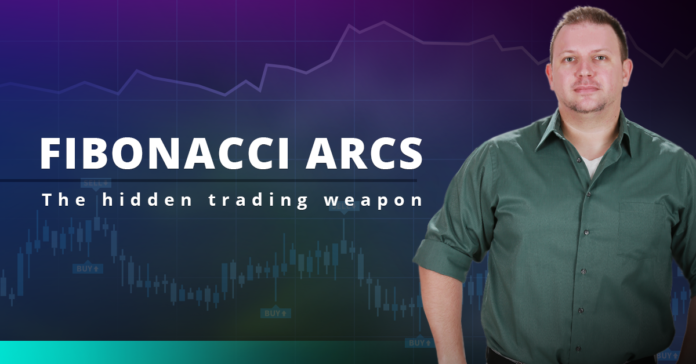Contents

## Introduction to Fibonacci Arcs

Fibonacci Arcs are half circles that extend out from a trend line. The first and third arcs are based on the Fibonacci ratios .382 (38.2%) and .618 (61.8%), respectively. These numbers are often rounded to 38% and 62%. The middle arc is set at .50 or 50%. After an advance, Fibonacci Arcs are measured using a Base Line that extends from trough to peak. Arcs are drawn along this line with radii that measure .382, .50 and .618 of the Base Line. These arcs mark potential support or reversal zones to watch as prices pullback after the advance. After a decline, Fibonacci Arcs are used to anticipate resistance or reversal zones for the counter-trend bounce. This article will explain the Fibonacci ratios and provide examples using Fibonacci Arcs to project support and resistance.

## The Sequence and Ratios

This article is not designed to delve too deep into the mathematical properties behind the Fibonacci sequence and Golden Ratio. There are plenty of other sources for that detail. A few basics, however, will provide the necessary background for the most popular numbers. Leonardo Pisano Bogollo (1170-1250), an Italian mathematician from Pisa, is credited with introducing the Fibonacci sequence to the West. It is as follows:

0, 1, 1, 2, 3, 5, 8, 13, 21, 34, 55, 89, 144, 233, 377, 610……

The sequence extends to infinity and contains many unique mathematical properties:

• After 0 and 1, each number is the sum of the two prior numbers (1+2=3, 2+3=5, 5+8=13 8+13=21 etc…).
• A number divided by the previous number approximates 1.618 (21/13=1.6153, 34/21=1.6190, 55/34=1.6176, 89/55=1.6181). The approximation nears 1.6180 as the numbers increase.
• A number divided by the next highest number approximates .6180 (13/21=.6190, 21/34=.6176, 34/55=.6181, 55/89=.6179 etc….). The approximation nears .6180 as the numbers increase. This is the basis for the 61.8% retracement.
• A number divided by another two places higher approximates .3820 (13/34=.382, 21/55=.3818, 34/89=.3820, 55/=144=3819 etc….). The approximation nears .3820 as the numbers increase. This is the basis for the 38.2% retracement. Also, note that 1 – .618 = .382

1.618 refers to the Golden Ratio or Golden Mean, also called Phi. The inverse of 1.618 is .618. These ratios can be found throughout nature, architecture, art and biology. In his book, Elliott Wave Principle, Robert Prechter quotes William Hoffer from the December 1975 issue of Smithsonian Magazine:

….the proportion of .618034 to 1 is the mathematical basis for the shape of playing cards and the Parthenon, sunflowers and snail shells, Greek vases and the spiral galaxies of outer space. The Greeks based much of their art and architecture upon this proportion. They called it the golden mean.

## Calculation

• Base Line: A line from point A to point B
• First Arc: Radius = .382 of Base Line
• Second Arc: Radius = .500 of Base Line
• Third Arc: Radius = .618 of Base Line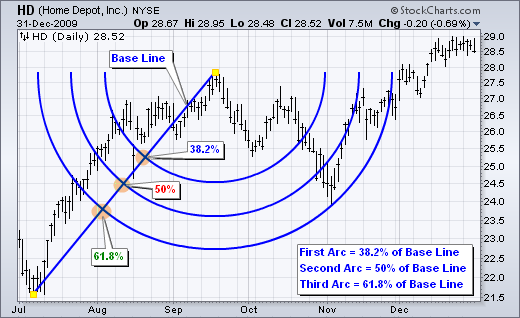The first step is to pick the peak and trough for the Base Line. Draw the line from trough to peak for an advance or from peak to trough for a decline. The example above shows Home Depot with a Base Line from trough to peak. The radius for the first Fibonacci Arc measures 38.2% of the Base Line. The radius for the second Fibonacci Arc is in the middle of the Base Line (50%). The radius for the third Fibonacci Arc measures 61.8% of the Base Line. Three half circles are drawn based on these radii. Notice how HD reversed near the third Fibonacci Arc (61.8%).

## Interpretation

Fibonacci Arcs add a time element to Fibonacci retracements. The Fibonacci Retracements Tool is based on a vertical line from trough to peak or from peak to trough. It is only concerned with the change in price. In contrast, a Base Line after an advance extends from trough to peak at an angle that is dependent on elapsed time (positive slope).

A Base Line after a decline extends from peak to trough at an angle that is also dependent on elapsed time (negative slope). The slope and length of the line depend on changes in both price and time. A big price movement over a long period of time produces a long Base Line with wide arcs. Conversely, a small price change over a short period of time produces a short Base Line with narrow arcs.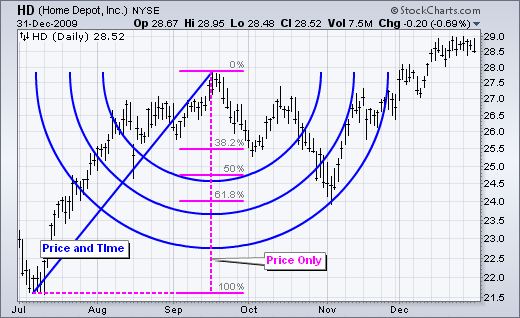Chart 2 shows Home Depot with the Fibonacci Retracements Tool and the Fibonacci Arcs. Both indicators extend from the July low to the September high. Notice how the Base Line slopes up and is longer than the vertical line in the Fibonacci Retracements Tool.

Whereas the Fibonacci Retracements Tool shows static retracement levels, the Fibonacci Arcs show dynamic retracements that evolve over time. Fibonacci Arcs drawn after a decline slowly work their way lower, which denotes falling resistance zones. Fibonacci Arcs drawn after an advance slowly work their way higher, which denotes rising support zones.

Despite these differences, Fibonacci Arcs are used the same way as the Fibonacci Retracements Tool. Both are used to anticipate support, resistance and reversals.

## Support Example

Chart 3 shows Nvdia (NVDA) with two Fibonacci Arcs marking support. The first Fibonacci Arcs extend from the February low to the May high. NVDA declined rather sharply from the early May high, but reversed course in between the 50% and 62% arcs.

The second Fibonacci Arcs (pink) extend from the May low to the June high. This advance is shorter so the Fibonacci Arcs are not as wide. NVDA moved lower in late June and early July, but found support near the 62% arc and moved sharply high in mid July.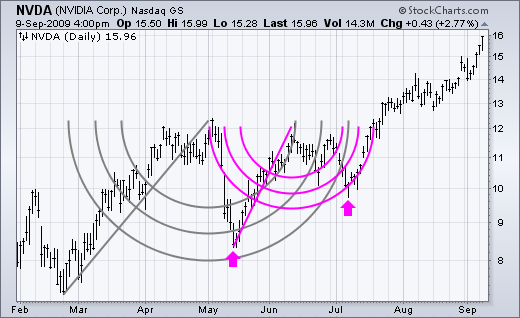Chart 4 shows Cleveland Cliffs (CLF) with a surge from March to May and then a choppy pullback. The stock bounced off the 38% arc in mid May and then bounced off the 62% arc in early July. Even traders that missed this bounce were given a second chance with the flag pullback and breakout in early September.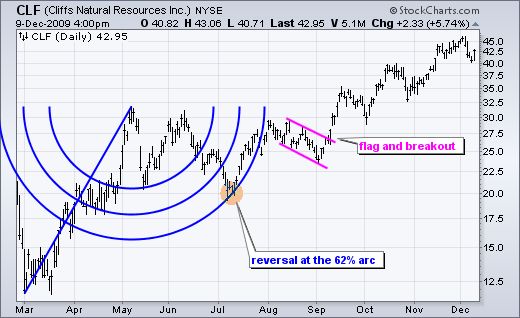Resistance Example

Chart 5 shows Darden Restaurants (DRI) with Fibonacci Arcs drawn from the April high to the May low. The stock rebounded at the end of May and hit resistance at the 62% arc in mid June. After a couple days stalling, the stock moved sharply lower with a long black candlestick. Resistance in the 44-45 area was confirmed by the May-June highs (orange box).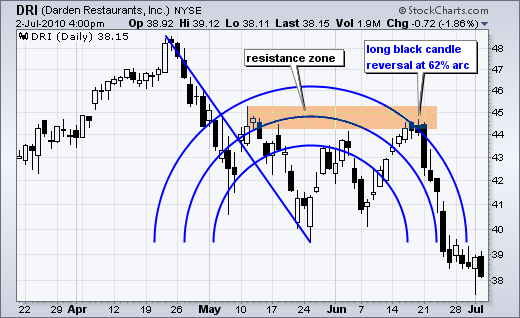Chart 6 shows Paychex (PAYX) with Fibonacci Arcs drawn from the May high to the July low (2008). The stock rebounded from mid July to mid August, but the advance hit resistance at the 62% arc. Also notice that a potentially bearish rising wedge formed. Even though the stock moved outside the 62% arc, this sideways move is not considered a bullish breakout. PAYX traded flat for a few days and then broke support to signal a continuation of the May-June decline.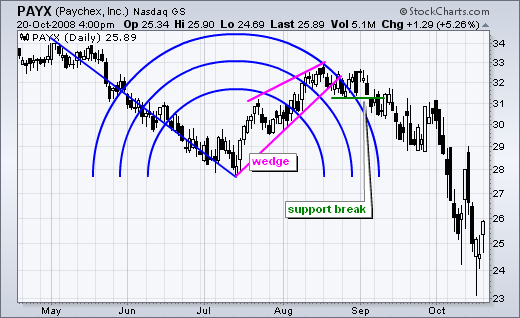Extending the Dateline

Chartists sometimes need to add extra time to see future support or resistance levels on the chart. Chart 7 shows the S&P 500 ETF (SPY) from mid March to mid July with 60 extra bars added. With these additional bars, chartists can see how the arcs evolve in the future. This can be done by going to “chart attributes” and entering the number of periods for the extension in the “extra bars” box.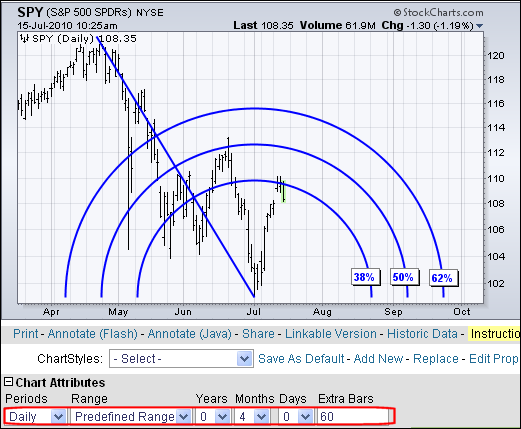Conclusions

Fibonacci Arcs are used to identify potential support, resistance or reversal points. As with the Fibonacci Retracements Tool, these reversal points assume that the move is corrective in nature.

A pullback after an advance is deemed a correction that will find support above the beginning of the advance.

A bounce after a decline is deemed a counter trend rally that will hit resistance below the beginning of the decline. Fibonacci Arcs allow users to anticipate the ending points for these counter-trend moves.

Like all annotation tools, Fibonacci Arcs are not meant as a stand alone system.

Just because prices approach an arc does not mean they will reverse.

Prices move right through these arcs in many cases. No indicator is perfect.

This is why chartists must use other tools to confirm support, resistance, bullish reversals and bearish reversals.

Sources:  https://en.wikipedia.org | http://stockcharts.com/

## How to Invest in Amazon

• Amazon’s net a bullish 450% 5-year gain
• Amazon’s ROI is 6x’s the S&P 500 Index of 67%
• AMZN shares may hit +\$10,000 by mid-November 2024
• Amazon’s growth since IPO tops more than 12,000%

### Now is the time to invest in Amazon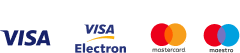Subscribe
Notify ofInline FeedbacksTheo
2 years ago

Hi Vladimir great article thanks. Is there a place where one can get hold of this indicator please.

02 years ago

Hi Theo

0Najam
2 years ago

Dear i love this indicator but i can’t find it can you give me this indicators template ?????

02 years ago

Hi Najam

02 years ago

Thank you guys!

0Cooper
4 years ago

Excellent aritcle

0Henry
4 years ago

Informative article, thank you for making it available

0